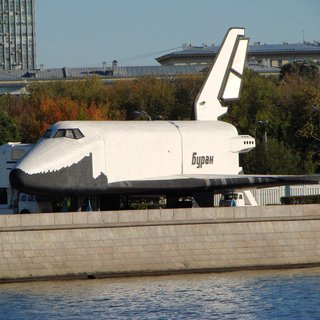OpenCV  3.3.0 Open Source Computer Vision

## Introduction

In this tutorial you will learn how to use opencv_dnn module for image classification by using GoogLeNet trained network from Caffe model zoo.

We will demonstrate results of this example on the following picture.Buran space shuttle

## Source Code

We will be using snippets from the example application, that can be downloaded here.

#include <opencv2/dnn.hpp>
using namespace cv;
using namespace cv::dnn;
#include <fstream>
#include <iostream>
#include <cstdlib>
using namespace std;
/* Find best class for the blob (i. e. class with maximal probability) */
static void getMaxClass(const Mat &probBlob, int *classId, double *classProb)
{
Mat probMat = probBlob.reshape(1, 1); //reshape the blob to 1x1000 matrix
Point classNumber;
minMaxLoc(probMat, NULL, classProb, NULL, &classNumber);
*classId = classNumber.x;
}
static std::vector<String> readClassNames(const char *filename = "synset_words.txt")
{
std::vector<String> classNames;
std::ifstream fp(filename);
if (!fp.is_open())
{
std::cerr << "File with classes labels not found: " << filename << std::endl;
exit(-1);
}
std::string name;
while (!fp.eof())
{
std::getline(fp, name);
if (name.length())
classNames.push_back( name.substr(name.find(' ')+1) );
}
fp.close();
return classNames;
}
int main(int argc, char **argv)
{
String imageFile = (argc > 1) ? argv : "space_shuttle.jpg";
if (net.empty())
{
std::cerr << "Can't load network by using the following files: " << std::endl;
std::cerr << "prototxt: " << modelTxt << std::endl;
std::cerr << "caffemodel: " << modelBin << std::endl;
exit(-1);
}
if (img.empty())
{
std::cerr << "Can't read image from the file: " << imageFile << std::endl;
exit(-1);
}
Mat inputBlob = blobFromImage(img, 1, Size(224, 224),
Scalar(104, 117, 123)); //Convert Mat to batch of images
Mat prob;
for (int i = 0; i < 10; i++)
{
CV_TRACE_REGION("forward");
net.setInput(inputBlob, "data"); //set the network input
t.start();
prob = net.forward("prob"); //compute output
t.stop();
}
int classId;
double classProb;
getMaxClass(prob, &classId, &classProb);//find the best class
std::cout << "Best class: #" << classId << " '" << classNames.at(classId) << "'" << std::endl;
std::cout << "Probability: " << classProb * 100 << "%" << std::endl;
std::cout << "Time: " << (double)t.getTimeMilli() / t.getCounter() << " ms (average from " << t.getCounter() << " iterations)" << std::endl;
return 0;
} //main

## Explanation

Also you need file with names of ILSVRC2012 classes: synset_words.txt.

Put these files into working dir of this program example.

2. Read and initialize network using path to .prototxt and .caffemodel files
3. Check that network was read successfully
if (net.empty())
{
std::cerr << "Can't load network by using the following files: " << std::endl;
std::cerr << "prototxt: " << modelTxt << std::endl;
std::cerr << "caffemodel: " << modelBin << std::endl;
exit(-1);
}
4. Read input image and convert to the blob, acceptable by GoogleNet

if (img.empty())
{
std::cerr << "Can't read image from the file: " << imageFile << std::endl;
exit(-1);
}
Mat inputBlob = blobFromImage(img, 1, Size(224, 224),
Scalar(104, 117, 123)); //Convert Mat to batch of images

Firstly, we resize the image and change its channel sequence order.

Now image is actually a 3-dimensional array with 224x224x3 shape.

Next, we convert the image to 4-dimensional blob (so-called batch) with 1x3x224x224 shape by using special cv::dnn::blobFromImages constructor.

5. Pass the blob to the network

net.setInput(inputBlob, "data"); //set the network input

In bvlc_googlenet.prototxt the network input blob named as "data", therefore this blob labeled as ".data" in opencv_dnn API.

Other blobs labeled as "name_of_layer.name_of_layer_output".

6. Make forward pass
prob = net.forward("prob"); //compute output
During the forward pass output of each network layer is computed, but in this example we need output from "prob" layer only.
7. Determine the best class
int classId;
double classProb;
getMaxClass(prob, &classId, &classProb);//find the best class
We put the output of "prob" layer, which contain probabilities for each of 1000 ILSVRC2012 image classes, to the prob blob. And find the index of element with maximal value in this one. This index correspond to the class of the image.
8. Print results